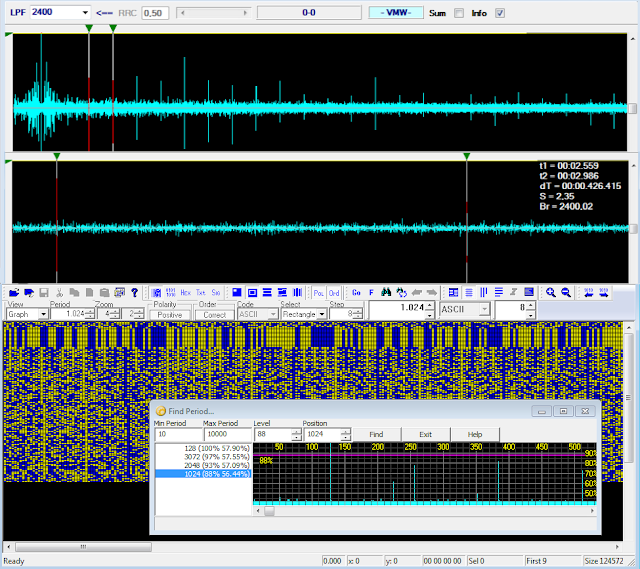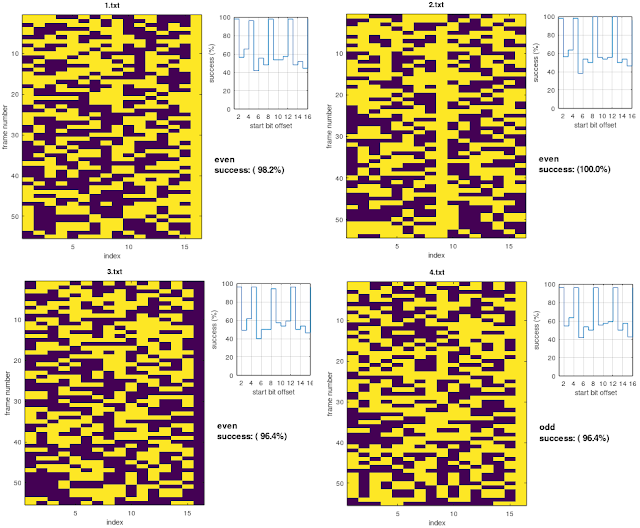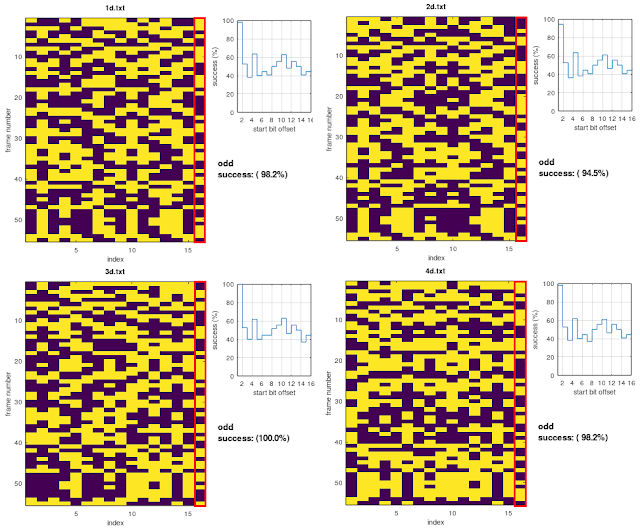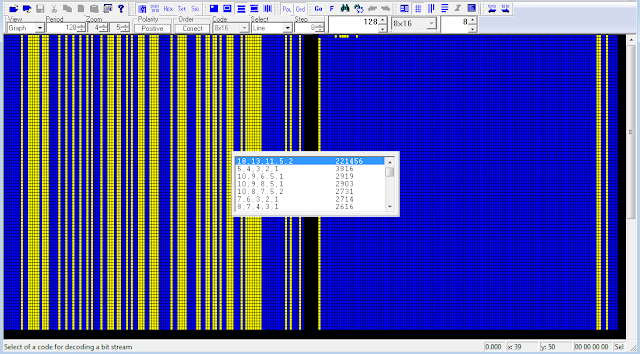## 15 December 2021

### Chinese PSK2 2400Bd serial waveform

This is my follow-up to an interesting post discussed on the radioscanner.ru forum about a PSK2 transmission of the Chinese Navy and started by my friend KarapuZ .  The raw demodulated stream consists of an initial preamble followed by data block consisting of a serie of 16-bit structures which are delimited by solid columns of "1"s or "0"s; the  period is calculated in 3072, 2048, and 1024 bit (128-bit length is due to the preamble sequences): the percentage values in figure 1 indicate respectively  the average ACF value for the given period, followed by the real value of the ACF for that period). Although the value of 1024 bit is the third positive result, both ACF and CCF indicate this as the most likely, being the other two integer multiples of it (x3, x2): this way, the stream consists of 64 "channels", each consisting of 16 bit.Fig. 1

As noted by my friend Cryptomaster, the 15-bit information between the solid columns is parity-checked; thus, assuming the parity bit is added, as usual, after the data string, each row could consist of 14 bits for data (x) + 1 parity bit (p) + 1 delimiter bit (d): xxxxxxxxxxxxxxpd.

I proceeded to the parity check of some channels taking into account all sixteen bits, given that:

* the add of a column of "0"s does not affect the parity checksum of the channel (even or odd);
* the add of a column of "1"s switches the parity checksum (from even to odd and vice-versa).

The results for some channels are shown in figure 2, it's to be noticed that the max number of dd/even parity parity matches occurs after shifted the stream (offset >1). Looking at the sequence of the parity checksums of the examined channels, and considering the previous assumptions, we get an apparently random alternation of odd and even parity checksums.Fig. 2

After the differential decoding, the channels' "delimiters" disappear, so I tried to get the differential decoding of each channel resorting to some sort of workaround: basically I cut off the 16-bit channels from the plain decoding and then I differential decoded each of them. Yes I know, it's an hazard as it assumes that the channels are individually differential encoded (in real-world the first bit of the n channel depends on the last bit of the n-1 channel) ... however, in that way, the channels are all odd-parity checked and perfectly aligned (figure 3).Fig. 3 - channels after their individual differential decoding

Since the use of a single parity bit cannot correct any errors, and given the amount of data transmitted, my idea is that they could use a kind of (16,k) coding with the overall parity bit added at the end of the codeword.

For what concerns the preamble sequence, it can be successfully descrambled using the polynomial x^18+x^13+x^11+x^5+x^2+1 (figure 4): it means that the preamble actually consists of a 128-bit pseudo-random sequence (PRBS) which is part of the M-sequence generated by the aforedmentioned polynomial.Fig. 4 - 128-bit PRBS used as preamble

1.What are the RF frequencies?

1.Heard on 10990,5 and 11104,5 (kHz, USB)

2.Ok thanks, here's an EE translation of footnote :

3.Yep, I'm "ansanto" there :)

2.Hi Antonio,

1.